# Triangulation algorithm for video games

Hi,

Today, I want to present you my Triangulation algorithm.

There is solutions on Internet, so Why? Because, I cannot find best suited solution I need. Maybe I didn’t dig enough, but clearly, my solution works 🙂
It is actually used in Alone In The Dark and Test Drive Unlimited games on PS3, XBOX360 and PC…

Ok, what is a Triangulation algorithm?
Well, in my case, I have a plane shape with multiple points and I want to draw this shape in a 3D game.
Condition: alls points must be in the final shape.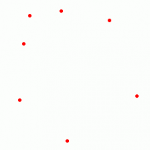Our graphics cards only know how to draw triangles. So I need to make Triangles, but how?

As you see, there is a lot’s of solutions:

What is the worst, the best?

I have a personal advice: in a 3D games, there is always little visuals artifacts on the triangle’s edges… Edges are difficult to keep clean. So my solution decreases the edge length.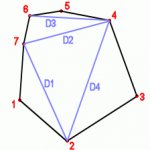Here,  edges length is = D1 + D2 + D3 + D4

# Here is my algorithm:

Put all the external edges length in an array indexed by the number of point :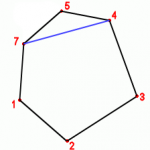For example, this is the edge of the point 5, so Array = D5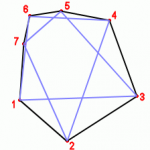Calculate all these edges lengthHere is the smallest : the edge of the point 6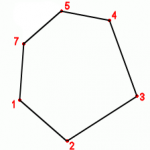Remove this point of your array and recompute the edge length of point 5 and 7 (no need to recompute all).Restart to find the smallest edge length and remove it…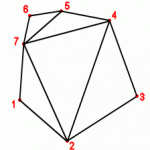When you have finished, your triangulation is done

My algorithm is very fast and very simple… The result of Delaunay’s Triangulation is better for optimizing surfaces, but mine is better for edge length.

For concave polygon, you just need to set an infinite edge length when the point is inside. Let me know in comment if you need more about it.

That’s all for today.

ZeroKcm

## 5 thoughts on “Triangulation algorithm for video games”

1.Taffa on a dit :

How does this algorithm work with arbitrary points (i.e, non-polygonal set of points) ?

•ZeroKcm on a dit :

It works very well and fast.

There is only one particulary case to check if the set of points is specific.

How is your set of points?

•ZeroKcm on a dit :

Unfortunatelly, this algorithm works only if you have the final shape.
But, you can find the good shape with a simple ConvexHull algorithm.
http://en.wikibooks.org/wiki/Algorithm_Implementation/Geometry/Convex_hull/Monotone_chain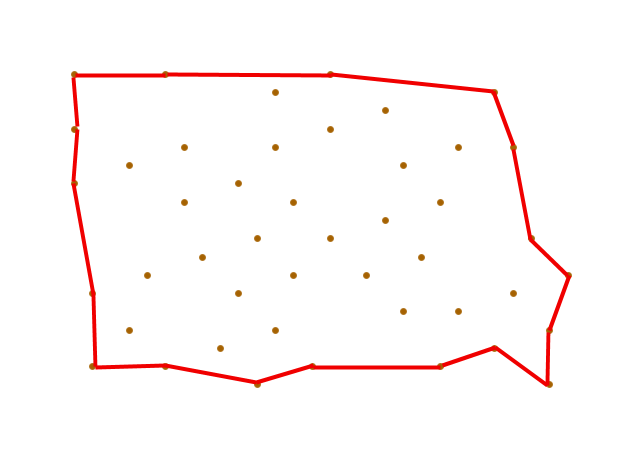If you really want to use all points in the shape you’ll have this type of result, but I’m pretty sure it’s not what you need 🙂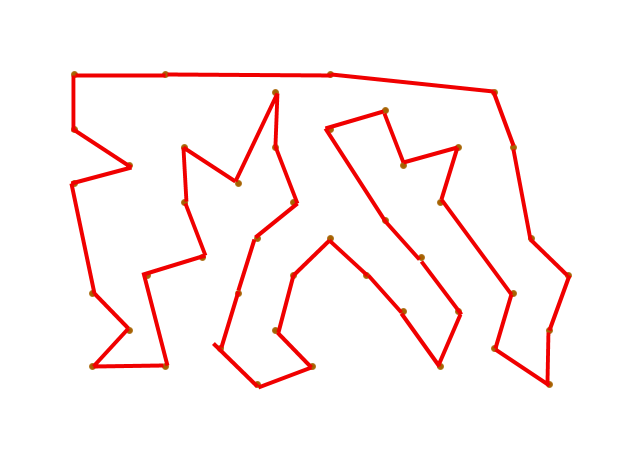•Taffa on a dit :

OK. Thanks, I understand better the « Condition: alls points must be in the final shape. »